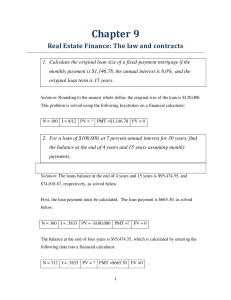# REAE 3325: Chapter 9 Real Estate Finance: The law and contracts. Here Lists the most tested Q&As on The law and contracts. All Explained.

PLDZ-41 In Stock
\$ 11.00 USD
Description

CLICK ON THE PRODUCT INFORMATION URL ABOVE FOR MORE

Chapter 9 Real Estate Finance: The law and contracts 1. Calculate the original loan size of a fixed-payment mortgage if the monthly payment is \$1,146.78, the annual interest is 8.0%, and the origina l loan term is 15 years. 2. For a loan of \$100,000, at 7 percent annual interest for 30 years, find the balance at the end of 4 years and 15 years assuming monhly payments. : 3. Consider a \$75,000 mortgage loan with an annual interest rate of 8%. The loan term is 7 years, but monthly payments will be based on a 30-year amortization schedule. What is the monthly payment? What will be the required balloon payment at the end of the loan term? The balloon payment at the end of year seven is \$69,358.07, as calculated with the following calculator keystrokes: 4. A mortgage banker is originating a level-payment mortgage with the following terms: Annual interest rate: 9 percent Loan term: 15 years Payment frequency: monthly Loan amount: \$160,000 Total up-front financing costs (including discount points): \$4,000 Discount points to lender: \$2,000 a. Calculate the annual percentage rate (APR) for Truth-in-Lending purposes. b. Calculate the lender’s yield with no prepayment. c. Calculate the lender’s yield with prepayment is five years. d. Calculate the effective borrowing costs with prepayment in five years.   a. The lender’s yield to maturity is 9.22 percent b. In order to calculate the lender’s yield, the loan balance remaining at the end of year five must first be calculated” The remaining balance is \$128,108.67. With this information, the lender’s yield is 9.34 percent as calculated below: c. The effective borrowing cost with prepayment in five years is 9.69 percent. 5. Suppose a one-year ARM loan has a margin of 2.75, an initial index of 3.00 percent, a teaser rate for the first year of 4.00 percent, and a cap of 1.00 percent. If the index rate is 3.00 percent at both the beginning and the end of the first year, what will be the interest rate on the loan in year two? If there is more than one possible answer, what does the outcome depend on? 6. Assume the following for a one-year rate adjustable rate mortgage loan that is tied to the one-year Treasury rate: Loan amount: \$150,000 Annual rate cap: 2% Life-of-loan cap: 5% Margin : 2.75% First-year contract rate: 5.50% One-year Treasury rate at end of year 1: 5.25% One-year Treasury rate at end of year 2: 5.50% Loan term in years: 30 Given these assumptions, calculate the following: a. Initial monthly payment b. Loan balance end of year 1 c. Year 2 contract rate d. Year 2 monthly payment e. Loan balance end of year 2 f. Year 3 contract rate g. Year 3 payment a. The loan balance at the end of year one is \$147,979, as shown below: b. Assuming the annual cap applies to the teaser rate, the interest rate in year two is 5.50 plus 2.00, or 7.50 percent. c. With a remaining term of 29 years, interest rate of 7.5 percent and a balance of \$147,979.41, the new payment in year 2 is \$1,044.32, calculated on a financial calculator with the following keystrokes. d. The loan balance at the end of year two is \$146,496: e. The year three contract interest rate is index plus margin, or 8.25 percent. f. The year three payment, based on a balance of \$146,496, remaining term of 28 years and an interest rate of 8.25 percent, is \$1,119. 7. Assume the following: Loan Amount: \$100,000 Interest rate: 10 percent annually Term: 15 years, monthly payments What is the monthly payment? What will be the loan balance at the end of nine years? What is the effective borrowing cost on the loan if the lender charges 3 points at origination and the loan goes to maturity? What is the effective borrowing cost on the loan if the lender charges 3 points at origination and the loan is prepaid at the end of year 9? a. The effective borrowing cost of the loan, with financing costs of 3 points, that is prepaid at the end of year nine: 10.61% (0.8839*12) 8. For a 30-year loan with a face value of 150,000 , 8 percent annual interest, and monthly payments find the remaining mortgage balance at the end of year 5 and 20. The monthly payment = 1,100.647 Loan balance at the end of year 5 =\$142,604.79 Loan balance at the end of year 20 = \$90,716.94 9. Consider a 20-year loan with a monthly payment of \$1,050.00 and an annual interest rate of 8.5 percent. What was the original loan size? Original loan size = \$120,992.38 10. You are considering buying a \$200,000 house with a 5 percent down payment, a 30-year mortgage, a fixed annual rate of 7.5 percent, and monthly payments. What is the annual payment? 11. Consider a \$200,000 mortgage loan with an annual interest rate of 7 percent. The loan term is 8 years but the monthly payment is based on a 25-year amortization period. Find the monthly payment and the balloon payment at the end of the loan term.   12. Assume a \$175,000 mortgage loan and 10-year term. The lender is charging an annual interest rate of 6 percent and 4 discount points at origination. What is the monthly payment assuming that it based on an amortization period of 30

Recent Reviews Write a Review
0 0 0 0 reviews